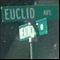Discussion about math, puzzles, games and fun.   Useful symbols: ÷ × ½ √ ∞ ≠ ≤ ≥ ≈ ⇒ ± ∈ Δ θ ∴ ∑ ∫  π  -¹ ² ³ °

You are not logged in.

## #1 2006-06-11 19:14:30

renjer
Member
Registered: 2006-04-29
Posts: 50

### Is there any easy way to calculate Principal Unit Normal Vectors?

Unit Tangent is easy to get. But when I get to unit normal, it kinda seems too difficult sometimes, particularly when you're trying to find T'.

Or when I try to use the tangential and normal accelerations (a=aT'+v^2KN) formula, that can get very hard too. (where a is the acceleration, T is the unit tangent vector, v is the velocity, K is the curvature and N is the unit normal).

So does anyone know a simpler way of doing this?

Offline

## #2 2006-06-12 13:22:26

Ricky
ModeratorRegistered: 2005-12-04
Posts: 3,791

### Re: Is there any easy way to calculate Principal Unit Normal Vectors?

Can you define "principal unit normal"?  I can't seem to find a definition on mathworld or any other place.

"In the real world, this would be a problem.  But in mathematics, we can just define a place where this problem doesn't exist.  So we'll go ahead and do that now..."

Offline

## #3 2006-06-12 15:33:04

renjer
Member
Registered: 2006-04-29
Posts: 50

### Re: Is there any easy way to calculate Principal Unit Normal Vectors?

I think this is the place where I found it.

http://www.ltcconline.net/greenl/course … annorm.htm

Offline